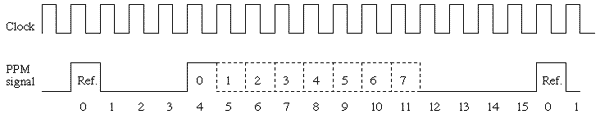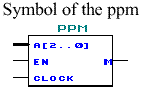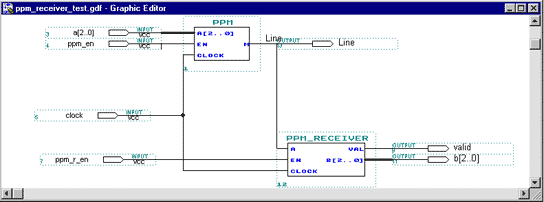## 1.2. Example in VHDL : PPM emitter and PPM receiver

### 1.2.1. The PPM signal

PPM (Pulse Position Modulation) is a serial data transmission protocol.

The PPM signal is composed of a reference pulse followed, a few clock cycles later, by another pulse, the width of this second pulse gives the value sent by the emitter.The advantage of the PPM signal is that the transmission is very reliable: due to the form of the signal an error can be detected very easily. This signal is by the way ideal for use in a very noisy environment.

The disadvantage is that the signal use a huge amount of band-width, in our example it¹s using 16 clock cycles for a 3 bit value, whereas others serial transmissions could use less than 6 clock cycles.

All this explain why the PPM transmission is chosen for most of the infra red remote controllers like television or CD-player remote controller.

### 1.2.2. The PPM emitter

The PPM emitter receive 3 input signals: a 3 bit integer (the value to transmit), an enabler, and the clock. There is only one output which is the PPM signal itself.

This is declared very simply in the entity declaration of the PPM emitter:

ENTITY ppm ISPORT(
a     : IN BIT_VECTOR (2 DOWNTO 0);
en    : IN BIT;
clock : IN BIT;
m     : OUT BIT);
END ppm;

The PPM signal is very simple to implement in VHDL, the process is only a 16 clock cycle loop which generates the reference pulse at first, and sets the signal to one at the fourth clock cycle, and finally reset it to zero when the input value, here b, is reached.

Here the value is stored in b which is an integer, whereas a is a vector of 3 bits. The VHDL language is very strict and does not allow you to operate tests between different types of signals or variables. That¹s why b is used here instead of a directly.

PROCESS (clock)
VARIABLE n : INTEGER RANGE 0 TO 15 := 0;
BEGIN
IF clock = '1' THEN
IF en = '1' THEN
IF n = 15 THEN
n := 0;
ELSE
IF   n = 0 THEN m <= '1'; -- Reference
ELSIF n = 1 THEN m <= '0'; -- Wait until 4
ELSIF n = 4 THEN m <= '1'; -- Set to '1'
ELSIF n > 4 THEN -- Until a value
IF (n - 4) > b THEN
m <= '0';
END IF;
END IF;
n := n + 1;
END IF;
ELSE -- en = '0'
n := 0;
m <= '0';
END IF;
END IF;
END PROCESS;

The PPM receiver is a little bit more complex than the emitter. This time the signal must be recognised instead of being created. The method used here to recognise the signal is to store the signal in a 16 bit memory, which is the length of the signal. This memory is compared to the possible formats of the signal, and when the signal match, we set the corresponding value to the output.

The PPM receiver have three input signal : the PPM signal itself, an enabler, and the clock. This time we use 2 outputs : a 3 bit integer which contain the result of the last match (the transmitted value), and a validation signal, this last signal is necessary because it announces when the signals match and so is useful to know if the output value of the receiver is the value of the current signal or only the stored value of the last signal. It can be used too as a flag to check if the transmission occurs and is correct.

ENTITY ppm_receiver ISPORT(
a : IN STD_LOGIC;
en : IN STD_LOGIC;
clock : IN STD_LOGIC;
val : OUT STD_LOGIC;
b : OUT STD_LOGIC_VECTOR (2 DOWNTO 0)
);

As previously said, we compare a stored signal to the possible formats. If the signal is not a possible signal, nothing occurs, but if it matches, the corresponding value is send to the output, and the val (validate) signal is set to one to announce that a PPM signal has been successfully received.

SIGNAL mem : STD_LOGIC_VECTOR (0 TO 15) := "0000000000000000";
BEGIN
PROCESS (clock)
BEGIN
IF clock = '1' THEN
FOR i IN 0 TO 14 LOOP
mem(i) <= mem(i+1);
END LOOP;
mem(15) <= a; IF en = '1' AND mem(0 TO 4) = "10001" AND mem (12 TO 15) = "0000" THEN
CASE mem(5 TO 11) IS
WHEN "0000000" =>
val <= '1';
b <= "000";
WHEN "1000000" =>
val <= '1';
b <= "001";
. we show only the beginning and the
. end for space reasons
WHEN "1111111" =>
val <= '1';
b <= "111";
WHEN OTHERS => val <= '0';
END CASE;
ELSE -- en = '0'
val <= '0';
END IF;
END IF;
END PROCESS;
END stdlogic;

### 1.2.4. Linking the components together

Now we have two components : a PPM emitter and a PPM receiver, but they are useless if we cannot link them together.

The VHDL allows us to use a VHDL component as a sub-component of another VHDL component. In order to use them we have to declare the component with their PORT assignment for the inputs and outputs. This is made just after the ARCHITECTURE statement.

ARCHITECTURE stdlogic OF ppm_fpga IS
COMPONENT ppm IS
PORT(
a, en, clock : IN BIT;
m : OUT BIT);
END COMPONENT;
FOR ALL : ppm USE ENTITY work.ppm(ver_max);
PORT(
a, en, clock : IN STD_LOGIC;
val : OUT STD_LOGIC;
b : OUT STD_LOGIC_VECTOR (2 DOWNTO 0));
END COMPONENT;
FOR ALL : ppm USE ENTITY work.ppm(ver_max);

After the declaration of the components, we don¹t use a process but set a label followed by the signal assignment to the ports of the components, i.e. the port mapping. Internal signals have to be declared to link the component together.

SIGNAL line, valid : STD_LOGIC; SIGNAL a, b : STD_LOGIC_VECTOR(2 DOWNTO 0);
BEGIN
p0 : ppm PORT MAP(a, pba, clock, line);
P1 : ppm_receiver PORT MAP(line, pbb, clock, valid, b);

The result is equivalent to the result we could obtain with a schematic editor.##### [ Contents | Previous Page | Next Page ]
For any comment/Pour tout commentaire : stephane@odul.net